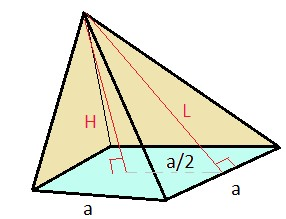# QOTD-Quantitative Aptitude- Mensuration

2019-07-21 | Team PendulumEdu

What will be the total surface area of the symmetrical pyramid having a square base with an area of 576 $$m^2$$ and height 5 $$m$$?

A) $${1200 m^2}$$

B) $$956 m^2$$

C) $$1050 m^2$$

D) $$1100 m^2$$

Solution

Given:

Height (H) = 5 m

Area of square base =$$576 m^2$$

So, Side of the square, a = $$\sqrt{576}$$ = 24 m

Now,The lateral height of the triangle, L

L = $$\sqrt{H^2+{(\frac{a}{2}})^2}$$

L = $$\sqrt{5^2+{(\frac{24}{2}})^2}$$

L = 13m

There are 4 symmetrical triangles in the pyramid so, the total surface area of the pyramid is

= 4 x (area of triangles) + area of base

= $$4*(\frac{1}{2}*base*height)+576$$

=$$4*(\frac{1}{2}*24*13)+576$$

= 624 + 576

$$1200 m^2$$

Hence, (a) is the correct answer.

Such type of question is asked in various government exam like SSC CGL, SSC MTS, SSC CPO, SSC CHSL, RRB JE, RRB NTPC, RRC GROUP D, RRB OFFICER SCALE-I, etc. Try free mock tests at PendulumEdu and learn easy techniques to handle typical mensuration questions.0